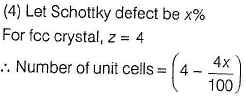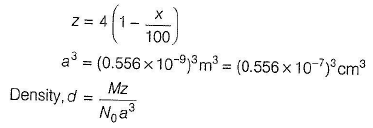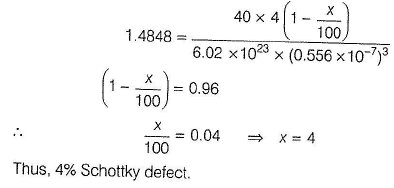Courses

# Test: Imperfection in Crystals

## 21 Questions MCQ Test Chemistry Class 12 | Test: Imperfection in Crystals

Description
This mock test of Test: Imperfection in Crystals for Class 12 helps you for every Class 12 entrance exam. This contains 21 Multiple Choice Questions for Class 12 Test: Imperfection in Crystals (mcq) to study with solutions a complete question bank. The solved questions answers in this Test: Imperfection in Crystals quiz give you a good mix of easy questions and tough questions. Class 12 students definitely take this Test: Imperfection in Crystals exercise for a better result in the exam. You can find other Test: Imperfection in Crystals extra questions, long questions & short questions for Class 12 on EduRev as well by searching above.
QUESTION: 1

### Only One Option Correct Type This section contains 9 multiple choice questions. Each question has four choices (a), (b), (c) and (d), out of which ONLY ONE is correct. Q.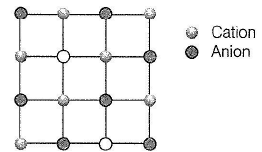Solution:

. (a) A Schottky defect consists of a pair of holes in the crystal lattice. One positive ion and one negative ion are absent.

Note This sort of defect occurs mainly in highly ionic compounds where cation and anion are of similar size, hence the coordination number is high (8.6) as in NaCI, CsCI, KCI, KBr

QUESTION: 2

### Structure shown in the figure represents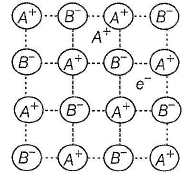Solution:

The picture is shown as cation excess that means metal excess with the absence of anion.

QUESTION: 3

### Which of the following defects is also known as dislocation defect?

Solution:

(a) If there is no deficie ncy of cation or anion but cation migrates to interstitial site, it is called dislocation. It is observed in the crystals with smaller cation and larger anion, so that cation can dislocate easily. It is called Frenkel defect.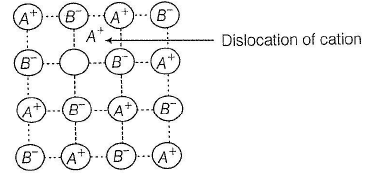QUESTION: 4

Cations are present in the interstitial sites in

Solution:

. (a) Frenkel defect is observed in the crystals in which cation is much smaller than the anion so that cation can migrate to interstitial position.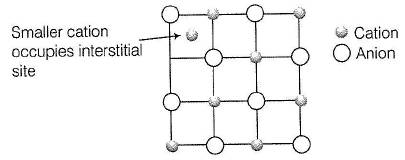QUESTION: 5

Select the correct statement about non-stoichiometric compounds

Solution:

(a)  Non-stoichiometric compounds are also called Berthollide compounds thus, correct.

(b) Since, composition of the constituent elements is not fixed, thus law of constant composition is not followed as is Fe 0,98 O thus, correct.

(c) If cation (say A+) is missing then, charge is balanced by the cation A2+. If anion (say B-) is missing, then charge is balanced by inserting electron (e-)thus, correct.

QUESTION: 6

In a face-centred cubic lattice, atom A occupies the corners of the cube and atom B occupies the face-centred positions. If one atom of B is missing from one of the face-centred points, the formula of the compound is

Solution: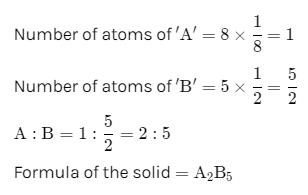QUESTION: 7

An element crystallises in fcc lattice having edge length 40 0 pm. Maximum radius of the atom which can be placed in the interstitial site without distorting the structure is

Solution:

(a) For fcc structure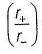= 0.414 for octahedral void

= 0.225 for tetrahedral void

Thus, maximum packing can be for octahedral void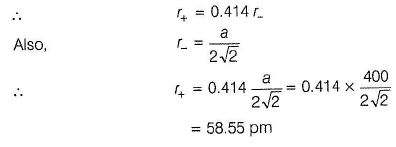QUESTION: 8

Experimentally it was found that a metal oxide has formula M0.98O. Metal M, is present as M2+ and M3+ in its oxide. The fraction of the metal which exists as M3+ would be:

Solution:

Metal oxide = M0.98O
If ‘x’ ions of M are in +3 state, then
3x + (0.98 – x) × 2 = 2
x = 0.04
So the percentage of metal in +3 state would be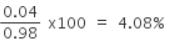QUESTION: 9

If NaCI is doped with 10-3 mole % of SrCI2 then , number of cation ic vacancies is

Solution:

(c)

Due to the addition of SrCI2, each Sr2+ ion replaces two Na+ ions, but occupies one Na+ lattice point. Thus, this exchange of Na+ ion by Sr2+ ion makes one cationic vacancy.

SrCI2 doped = 10-3 mol per 100 mol = 10-5 mol per 1 mol

∴ Cation vacancies = 10-5 mol per 1 mol

= 10-5 x N0 mol-1

= 10-5 x 6.02 x 1023

Total = 6.02 x 1018 cationic vacancies mol-1

*Multiple options can be correct
QUESTION: 10

One or More than One Options Correct Type
This section contains 7 multiple type questions. Each question has 4 choices (a), (b), (c) and (d), out of which ONE or MORE THAN ONE are correct.

Q.

AgBr(s) crystals can exhibit one or more types of defect which are

Solution:

(a, b) AgBr is a covalent c om pound with smaller A g+ (= 115 pm) and larger Br-(≈196 pm) thus, Ag+ ion can be migrated to interstitial site showing Frenkel defect. In AgBr, there is also Schottky defect per1016 ions.

QUESTION: 11

Substitution of foreign atom in the site of parent atom in crystal is a?

Solution:

In Substitution impurity, foreign atom takes the position of parent atom. Toatal number of atoms remains same after the defect.

QUESTION: 12

As grain size of metal increases, Strength of metal:

Solution:

Strength of metal is inversely proportional to size of grain. Coarse are softer in nature while material having fine grain structure are hard in nature.

*Multiple options can be correct
QUESTION: 13

Select the correct statements about the given structure.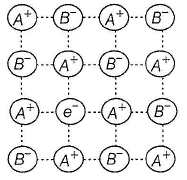Solution:

. (a,b,c,d)

(a) Since, number of cations > number of anions, hence it I represents metal-excess defect. It is similar to Schottky defect i in which equal number of cations and anions are missing | thus, correct.

(b) Charge is balanced by electrons, these are called F-centres (farbe, colour producing). Compounds with F-centres are ! coloured. Greater the number of F-centres, greater the ! intensity thus, correct.

(c) Due to the unpaired electrons these are paramagnetic thus, correct. j:

(d) When NaCI, KCl, etc., are strongly heated in flame, they become deficient in anions and represented as AS, _ s where, § is small fraction.
Non-stoichiometric form of NaCl is yellow and that is KClis blue-lilac thus, correct

*Multiple options can be correct
QUESTION: 14

Select the correct statement(s) about following structure.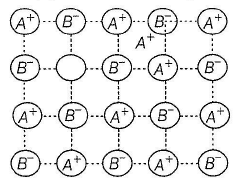Solution:

(a) correct
(b) As cation (A' ) occupies interstitial position, thus cation is smaller than anion, thus correct.
(c) Due to the smaller cation and larger anion, coordination number Is usually low (4, 6), thus correct.
(d) By Fajans' rule, smaller cations polarise larger anions thus, compounds are covalent, thus correct.

*Multiple options can be correct
QUESTION: 15

Which of the following statement(s) is/are correct?

Solution:

(a, b)

(a) When cation, anion or both are missing, molar mass is y ass decreased. Since, density = Mass/ Volume. hence density Volume decreases, thus correct.

(b) When there is insertion of cation (A + ) in the interstitial site, it causes metal-excess defect. Thus, molar mass increases, hence density increases, thus correct.

(c) Presence of impurity increases molar mass (as doping of metal with P or Si), hence density changes, thus incorrect.

(d) In Frenkel defect, cation is dislocated without affecting molar mass thus, no change in density , thus correct

QUESTION: 16

Comprehension Type
This section contains a passage describing theory, experiments, data, etc. Two questions related to the paragraph have been given. Each question has only one correct answer out of the given 4 options (a), (b), (c) and (d).

Passage I

The crystal AB (rock salt structure) has m ole cular w eight 6.02 y u, where y is an arbitrary unit in u. Also, given minimum distance between cation and anion = y 1/3 nm and observed density = 20 kg m-3.

Q.

Theoretical density of AB is

Solution:

(b)

AB is rock salt like structure, thus number of AB molecule per
unit cell = 4, a = 2y1/3 nm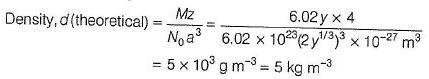Obseved value of d(20 kgm-3) >>5kgm-3

Thus, there is excess of metal ions which incerease mass hence the density

QUESTION: 17

Passage

The crystal AB (rock salt structure) has m ole cular w eight 6.02 y u, where y is an arbitrary unit in u. Also, given minimum distance between cation and anion = y 1/3 nm and observed density = 20 kg m-3.

Q.

Defect present in the crystal is of the type

Solution:

AB is rock salt like structure, thus number of AB molecules per unit cell  =4, a = 2y1/3 nm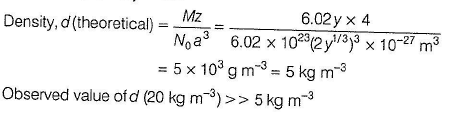Thus, there is excess of metal ions which increases mass hence the density.

QUESTION: 18

Passage II

Titanium (II) oxide has a rock salt structure. X-ray diffraction data shows that the length of one edge of the cubic unit cell for TiO with 1:1 ratio of Ti to O is 418 pm and the density is 4.92 g cm-3. ( Atomic mass of Ti = 48).

Q.

Defect present in the crystal is of the type

Solution:

Rock salt (NaCl) is fcc type structure, z = 4

M = 64 g mol-1, a = 418 pm

theoretical density (d) =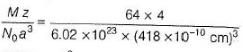= 5.82 g cm-3

Experimental density = 4.92 g cm-3

thus, experimental density << theoretical density

thus, there is vacancy in the crystal

d= Mass / Volume

Due to decrease in density, molar mass should decrease. this indicates Schottky defect.

Let Schottky defect be x%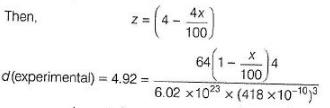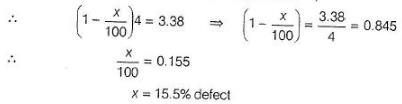QUESTION: 19

Passage II

Titanium (II) oxide has a rock salt structure. X-ray diffraction data shows that the length of one edge of the cubic unit cell for TiO with 1:1 ratio of Ti to O is 418 pm and the density is 4.92 g cm-3. ( Atomic mass of Ti = 48).

Q.

% of vacancies (defects) in the structure is

Solution:

Rock salt (NaCl) is fcc type structure, z = 4

M = 64 g mol-1, a = 418 pm

theoretical density (d) == 5.82 g cm-3

Experimental density = 4.92 g cm-3

thus, experimental density << theoretical density

thus, there is vacancy in the crystal

d= Mass / Volume

Due to decrease in density, molar mass should decrease. this indicates Schottky defect.

Let Schottky defect be x%QUESTION: 20

Matching List Type
Choices for the correct combination of elements from Column I and Column II are given as options (a), (b), (c) and (d), out of which one is correct.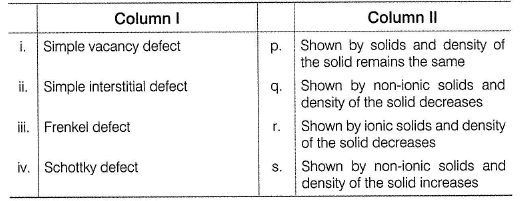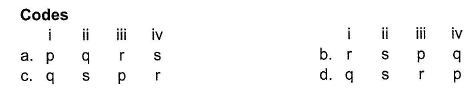Solution:

(i) When cation or anion is absent, molar mass decreases hence, density decreases.
Thus, (i) - » (q)

(ii) When cation is packed in the interstitial site, molar mass increases. Hence, density increases.
Thus, (ii) - » (s)

(iii) When smaller cation migrates into the interstitial site, Frenkel defect is observed. There is no change in molar mass and thus, no change in density.
Thus, (iii) -> (p)

(iv) As in NaCI, when cation and anion are missing (Schottky defect), molar mass and thus, density decreases.
Thus, (iv) - 0

*Answer can only contain numeric values
QUESTION: 21

One Integer Value Correct Type
This section contains 1 question, when worked out will result in an integer value from 0 to 9 (both inclusive)

Q.

Calcium crystallises in a face-centred cubic unit cell with a = 0.556 nm and density 1.4848 g/cm3. Percentage of Schottky defects in this crystal is ... .

Solution: